# NCERT Solutions for Class 12 Chemistry chapter 4-Chemical Kinetics

## NCERT Solutions for Class 12 Chemistry

NCERT Solutions for class-12 Chemistry Chapter 4 Chemical Kinetics is prepared by our senior and renowned teachers of Physics Wallah primary focus while solving these questions of class-12 in NCERT textbook, also do read theory of this Chapter 4 Chemical Kinetics while going before solving the NCERT questions. Our Physics Wallah team Prepared Other Subjects NCERT Solutions for class 12.

### Answer the following Questions.

Question1. For the reaction R → P, the concentration of a reactant changes from 0.03 M to 0.02 M in 25 minutes. Calculate the average rate of reaction using units of time both in minutes and seconds.

Solution :
Average rate of reaction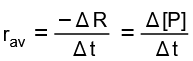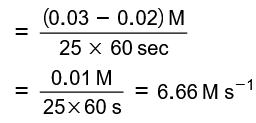= 6.67 × 10−6 M s−1

Question2. In a reaction, 2A → Products, the concentration of A decreases from 0.5 mol L−1 to 0.4 mol L−1 in 10 minutes. Calculate the rate during this interval?

Solution :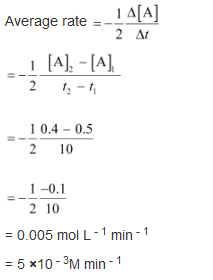= 0.005 mol L−1 min−1

= 5 × 10−3 M min−1

Question3. For a reaction, A + B → Product; the rate law is given by, r  = k [A]½ [B]2. What is the order of the reaction?

Solution :
The order of the reaction r  = k [A]½ [B]2

= 1 / 2 + 2

= 2.5

Question4. The conversion of molecules X to Y follows second order kinetics. If concentration of X is increased to three times how will it affect the rate of formation of Y?

Solution :
The reaction X → Y follows second order kinetics.

Therefore, the rate equation for this reaction will be:

Rate = k[X]2 (1)

Let [X] = a mol L−1, then equation (1) can be written as:

Rate1 = k .(a)2

= ka2

If the concentration of X is increased to three times, then [X] = 3a mol L−1

Now, the rate equation will be:

Rate = k (3a)2

= 9(ka2)

Hence, the rate of formation will increase by 9 times.

Question5. A first order reaction has a rate constant 1.15 10−3 s−1. How long will 5 g of this reactant take to reduce to 3 g?

Solution :
From the question, we can write down the following information:

Initial amount = 5 g

Final concentration = 3 g

Rate constant = 1.15 10−3 s−1

We know that for a 1st order reaction,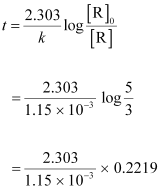= 444.38 s

= 444 s (approx)

Question6. Time required to decompose SO2Cl2 to half of its initial amount is 60 minutes. If the decomposition is a first order reaction, calculate the rate constant of the reaction.

Solution :
We know that for a 1st order reaction,

t½  = 0.693 / k

It is given that t1/2 = 60 min

k = 0.693 / t½

= 0.693 / 60

= 0.01155 min-1

= 1.155 min-1

Or

k = 1.925 x 10-2 s-1

Question7. What will be the effect of temperature on rate constant?

Solution :
The rate constant of a reaction is nearly doubled with a 10° rise in temperature. However, the exact dependence of the rate of a chemical reaction on temperature is given by Arrhenius equation,

k = Ae - Ea / RT

Where,

A is the Arrhenius factor or the frequency factor

T is the temperature

R is the gas constant

Ea is the activation energy

Question8. The rate of the chemical reaction doubles for an increase of 10 K in absolute temperature from 298 K. Calculate Ea.

Solution :
It is given that T1 = 298 K

∴T2 = (298 + 10) K

= 308 K

We also know that the rate of the reaction doubles when temperature is increased by 10°.

Therefore, let us take the value of k1 = k and that of k2 = 2k

Also, R = 8.314 J K−1 mol−1

Now, substituting these values in the equation: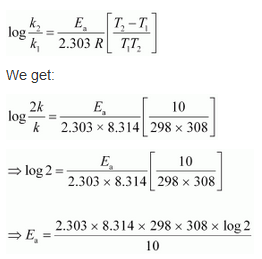= 52897.78 J mol−1

= 52.9 kJ mol−1

Question9. The activation energy for the reaction 2HI(g) → H2 + I2(g) is 209.5 kJ mol−1 at 581K. Calculate the fraction of molecules of reactants having energy equal to or greater than activation energy?

Solution :
In the given case:

Ea = 209.5 kJ mol−1 = 209500 J mol−1

T = 581 K

R = 8.314 JK−1 mol−1

Now, the fraction of molecules of reactants having energy equal to or greater than activation energy is given as:

x = e−Ea / RT

⇒Inx= −Ea / RT

⇒logx=−Ea / 2.303RT

⇒logx= −209500Jmol−1 / 2.303 × 8.314JK−1mol−1×581

=−18.8323

Now,x= Antilog (−18.8323)

=1.471×10−19

Question10. From the rate expression for the following reactions, determine their order of reaction and the dimensions of the rate constants.

(i) 3 NO(g) → N2O (g) Rate = k[NO]2

(ii) H2O2 (aq) + 3 I (aq) + 2 H+ → 2 H2O (l) + I3- Rate = k[H2O2][I]

(iii) CH3CHO(g) → CH4(g) + CO(g) Rate = k [CH3CHO]3/2

(iv) C2H5Cl(g) → C2H4(g) + HCl(g) Rate = k [C2H5Cl]

Solution :
(i) Given rate = k [NO]2

Therefore, order of the reaction = 2

Dimension of  k = Rate / [NO]2

= mol L-1 s-1 / (mol L-1)2

= mol L-1 s
-1 / mol2 L-2

= L mol-1s-1

(ii) Given rate = k [H2O2] [I]

Therefore, order of the reaction = 2

Dimension of

k = Rate  / [H2O2][I - ]

= mol L-1 s-1  / (mol L-1) (mol L-1)

= L mol-1 s-1

(iii) Given rate = k [CH3CHO]3/2

Therefore, order of reaction = 3 / 2

Dimension of  k = Rate / [CH3CHO]3/2

=  mol L-1 s-1   / (mol L-1)3/2

= mol L
-1   s-1     / mol3/2  L-3/2

= L½ mol-½  s-1

(iv) Given rate = k [C2H5Cl]

Therefore, order of the reaction = 1

Dimension of k = Rate /  [C2H5Cl]

= mol L-1 s-1   / mol L-1

= s-1

Question11. For the reaction:

2A + B → A2B

the rate = k[A][B]2 with k = 2.0 × 10−6 mol−2 L2 s−1. Calculate the initial rate of the reaction when [A] = 0.1 mol L−1, [B] = 0.2 mol L−1. Calculate the rate of reaction after [A] is reduced to 0.06 mol L−1.

Solution :
The initial rate of the reaction is

Rate = k [A][B]2

= (2.0 × 10−6 mol−2 L2 s−1) (0.1 mol L−1) (0.2 mol L−1)2

= 8.0 × 10−9 mol−2 L2 s−1

When [A] is reduced from 0.1 mol L−1 to 0.06 mol−1, the concentration of A reacted = (0.1 − 0.06) mol L−1 = 0.04 mol L−1

Therefore, concentration of B reacted 1/2 x 0.04 mol L-1 = 0.02 mol L−1

Then, concentration of B available, [B] = (0.2 − 0.02) mol L−1

= 0.18 mol L−1

After [A] is reduced to 0.06 mol L−1, the rate of the reaction is given by,

Rate = k [A][B]2

= (2.0 × 10−6 mol−2 L2 s−1) (0.06 mol L−1) (0.18 mol L−1)2

= 3.89 mol L−1 s−1

Question12. The decomposition of NH3 on platinum surface is zero order reaction. What are the rates of production of N2 and H2 if k = 2.5 × 10−4 mol−1 L s−1?

Solution :
The decomposition of NH3 on platinum surface is represented by the following equation.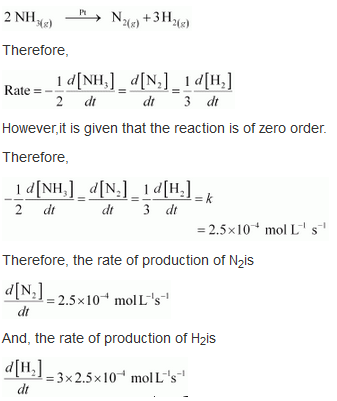= 7.5 × 10−4 mol L−1 s−1

Question13. The decomposition of dimethyl ether leads to the formation of CH4, H2 and CO and the reaction rate is given by

Rate = k [CH3OCH3]3/2

The rate of reaction is followed by increase in pressure in a closed vessel, so the rate can also be expressed in terms of the partial pressure of dimethyl ether, i.e.,

rate = k (PCH3OCH3)3/2

If the pressure is measured in bar andtime in minutes, then what are the units of rate and rate constants?

Solution :
If pressure is measured in bar and time in minutes, then

Unit of rate = bar min−1

Rate = k [CH3OCH3]3/2

⇒ k =Rate / [CH3OCH3]3/2

Therefore, unit of rate constants(k) = bar min−1 / bar3/2

= bar-½ min -1

Question14. Mention the factors that affect the rate of a chemical reaction.

Solution :
The factors that affect the rate of a reaction are as follows.

(i) Concentration of reactants (pressure in case of gases)

(ii) Temperature

(iii) Presence of a catalyst

15. A reaction is second order with respect to a reactant. How is the rate of reaction affected if the concentration of the reactant is

(i) doubled (ii) reduced to half?

Solution :
Letthe concentration of the reactant be [A] = a

Rate of reaction, R = k [A]2

= ka2

(i)If the concentration of the reactant is doubled, i.e. [A] = 2a, then the rate of the reaction would be

R = k(2a)2

= 4ka2

= 4 R

Therefore, the rate of the reaction would increase by 4 times.

(ii) If the concentration of the reactant is reduced to half, i.e. [A] = 1/2 a , then the rate of the reaction would be

R = k(1/2a)2

= 1/4 Ka2

= 1/4 R

Therefore, the rate of the reaction would be reduced to

16. What change would happen in the rate constant of a reaction when there is a change in its temperature? How can this temperature effect on rate constant be represented quantitatively?

Solution :
When a temperature of 10∘ rises for a chemical reaction then the rate constant increases and becomes near to double of its original value.

The temperature effect on the rate constant can be represented quantitatively by Arrhenius equation,

k=Ae−Ea/RT

Where,

k = rate constant,

A = Frequency factor / Arrhenius factor,

R = gas constant

T = temperature

Ea = activation energy for the reaction.

Question17. In a pseudo first order hydrolysis of ester in water, the following results were obtained:

 t/s 0 30 60 90 [Ester]mol L−1 0.55 0.31 0.17 0.085

(i) Calculate the average rate of reaction between the time interval 30 to 60 seconds.

(ii) Calculate the pseudo first order rate constant for the hydrolysis of ester.

Solution :
(i) Average rate of reaction between the time interval, 30 to 60 seconds, d[ester] / dt

= (0.31-0.17) / (60-30)

= 0.14 / 30

= 4.67 × 10−3 mol L−1 s−1

(ii) For a pseudo first order reaction,

k = 2.303/ t log [R]º / [R]

For t = 30 s, k1

= 1.911 × 10−2 s−1

For t = 60 s, k1 = 2.303/ 30 log 0.55 / 0.31

= 1.957 × 10−2 s−1

For t = 90 s,  k3 = 2.303/ 90 log 0.55 / 0.085

= 2.075 × 10 - 2s - 1

= 2.075 × 10−2 s−1

Then, average rate constant, k = k1 + k2+ k3  / 3

= 1.911 × 10 - 2  + 1.957 × 10 - 2 + 2.075 × 10 - 2 / 3

= 1.981 x 10-2 s - 1

Question18. A reaction is first order in A and second order in B.

(i) Write the differential rate equation.

(ii) How is the rate affected on increasing the concentration of B three times?

(iii) How is the rate affected when the concentrations of both A and B are doubled?

Solution :
(i) The differential rate equation will be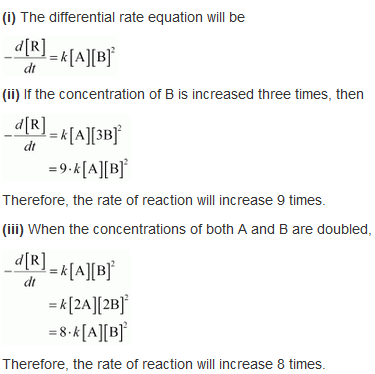Question19. In a reaction between A and B, the initial rate of reaction (r0) was measured for different initial concentrations of A and B as given below:

 A/ mol L−1 0.20 0.20 0.40 B/ mol L−1 0.30 0.10 0.05 r0/ mol L−1 s−1 5.07 × 10−5 5.07 × 10−5 1.43 × 10−4

What is the order of the reaction with respect to A and B?

Solution :
Let the order of the reaction with respect to A be x and with respect to B be y.

Therefore,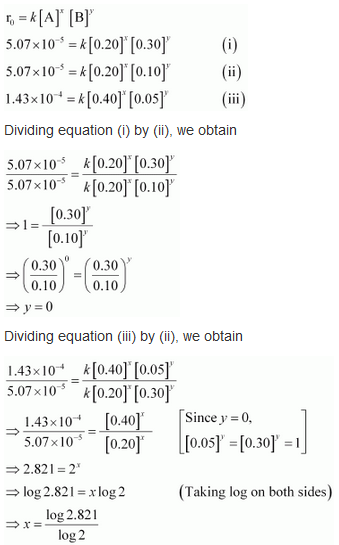Dividing equation (iii) by (ii), we obtain

= 1.496

= 1.5 (approximately)

Hence, the order of the reaction with respect to A is 1.5 and with respect to B is zero.

Question20. The following results have been obtained during the kinetic studies of the reaction:

2A + B → C + D

 Experiment A/ mol L−1 B/ mol L−1 Initial rate of formation of D/mol L−1 min−1 I 0.1 0.1 6.0 × 10−3 II 0.3 0.2 7.2 × 10−2 III 0.3 0.4 2.88 × 10−1 IV 0.4 0.1 2.40 × 10−2

Determine the rate law and the rate constant for the reaction.

Solution :
Let the order of the reaction with respect to A be x and with respect to B be y.

Therefore, rate of the reaction is given by,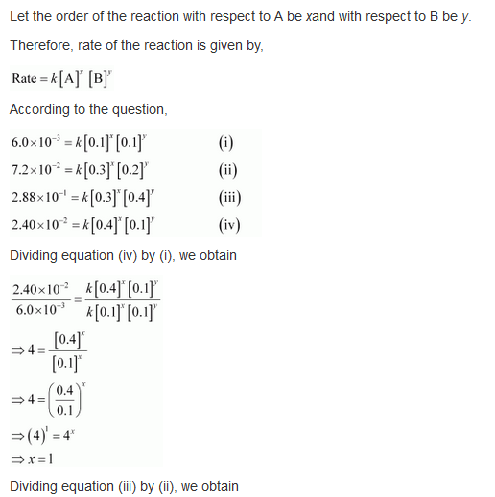Dividing equation (iv) by (i), we obtainDividing equation (iii) by (ii), we obtain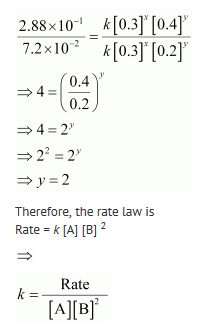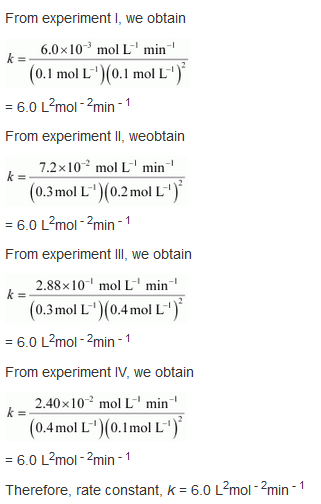Question21. The reaction between A and B is first order with respect to A and zero order with respect to B. Fill in the blanks in the following table:

 Experiment A/ mol L−1 B/ mol L−1 Initial rate/mol L−1 min−1 I 0.1 0.1 2.0 × 10−2 II -- 0.2 4.0 × 10−2 III 0.4 0.4 -- IV -- 0.2 2.0 × 10−2

Solution :
The given reaction is of the first order with respect to A and of zero order with respect to B.

Therefore, the rate of the reaction is given by,

Rate = k [A]1 [B]0

⇒ Rate = k [A]

From experiment I, we obtain

2.0 × 10−2 mol L−1 min−1 = k (0.1 mol L−1)

⇒ k = 0.2 min−1

From experiment II, we obtain

4.0 × 10−2 mol L−1 min−1 = 0.2 min−1 [A]

⇒ [A] = 0.2 mol L−1

From experiment III, we obtain

Rate = 0.2 min−1 × 0.4 mol L−1

= 0.08 mol L−1 min−1

From experiment IV, we obtain

2.0 × 10−2 mol L−1 min−1 = 0.2 min−1 [A]

⇒ [A] = 0.1 mol L−1

Question22. Calculate the half-life of a first order reaction from their rate constants given below:

(i) 200 s−1 (ii) 2 min−1 (iii) 4 years−1

Solution :
(i) Half life, t 1/2 = 0.693 / k

= 0.693 / 200 s-1

= 3.47×10 -3 s (approximately)

(ii) Half life, t 1/2 = 0.693 / k

= 0.693 / 2 min-1

= 0.35 min (approximately)

(iii) Half life, t 1/2 = 0.693 / k

= 0.693 / 4 years-1

= 0.173 years (approximately)

Question23. The half-life for radioactive decay of 14C is 5730 years. An archaeological artifact containing wood had only 80% of the 14C found in a living tree. Estimate the age of the sample.

Solution :
Here,  k = 0.693 / t1/2

= 0.693 / 5730 years-1

It is known that,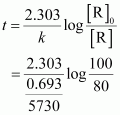= 1845 years (approximately)

Hence, the age of the sample is 1845 years.

Question24. The experimental data for decomposition of N2O5

[2N2O5 → 4NO2 + O2]  in gas phase at 318K are given below:

 t(s) 0 400 800 1200 1600 2000 2400 2800 3200 102 × [N2O5]  mol L-1 1.63 1.36 1.14 0.93 0.78 0.64 0.53 0.43 0.35

(i) Plot [N2O5] against t.

(ii) Find the half-life period for the reaction.

(iii) Draw a graph between log [N2O5] and t.

(iv) What is the rate law?

(v) Calculate the rate constant.

(vi) Calculate the half-life period from k and compare it with (ii).

Solution :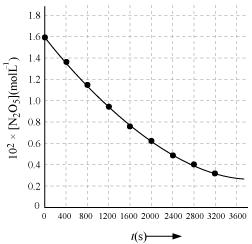(ii) Time corresponding to the concentration, 1630x102 / 2 mol L-1 = 81.5 mol L-1 is the half life. From the graph, the half life is obtained as 1450 s.

(iii)

 t(s) 102 × [N2O5]  mol L-1 Log[N2O5] 0 1.63 − 1.79 400 1.36 − 1.87 800 1.14 − 1.94 1200 0.93 − 2.03 1600 0.78 − 2.11 2000 0.64 − 2.19 2400 0.53 − 2.28 2800 0.43 − 2.37 3200 0.35 − 2.46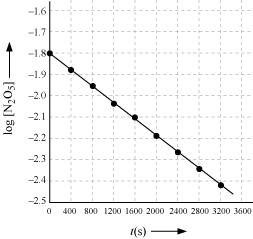(iv) The given reaction is of the first order as the plot, Log[N2O5v/s t, is a straight line. Therefore, the rate law of the reaction is

Rate = k [N2O5]

(v) From the plot, Log[N2O5] v/s t, we obtain

- k /2.303

Again, slope of the line of the plot  Log[N2O5v/s t is given by

- k / 2.303. = -0.67 / 3200

Therefore, we obtain,

- k / 2.303  = - 0.67 / 3200

⇒ k = 4.82 x 10-4 s-1

(vi) Half-life is given by,

t½ = 0.693 / k

= 0.639 / 4.82x10-4 s

=1.438 x 103

This value, 1438 s, is very close to the value that was obtained from the graph.

25. The rate constant for a first order reaction is 60 s−1. How much time will it take to reduce the initial concentration of the reactant to its 1/16th value?

Solution :
It is known that,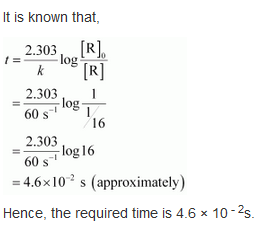Question26. During nuclear explosion, one of the products is 90Sr with half-life of 28.1 years. If 1μg of 90Sr was absorbed in the bones of a newly born baby instead of calcium, how much of it will remain after 10 years and 60 years if it is not lost metabolically.

Solution :
,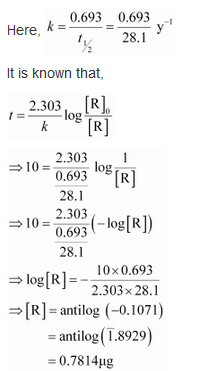Therefore, 0.7814 μg of 90Sr will remain after 10 years.

Again,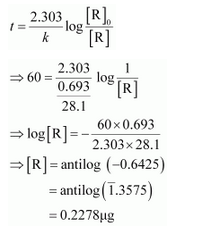Therefore, 0.2278 μg of 90Sr will remain after 60 years.

Question27. For a first order reaction, show that time required for 99% completion is twice the time required for the completion of 90% of reaction.

Solution :
For a first order reaction, the time required for 99% completion is

t1 = 2.303/k Log 100/100-99

= 2.303/k Log 100

= 2x 2.303/k

For a first order reaction, the time required for 90% completion is

t2 = 2.303/k Log 100 / 100-90

= 2.303/k Log 10

= 2.303/k

Therefore, t1 = 2t2

Hence, the time required for 99% completion of a first order reaction is twice the time required for the completion of 90% of the reaction.

Question28. A first order reaction takes 40 min for 30% decomposition. Calculate t1/2.

Solution :
For a first order reaction,

t = 2.303/k Log [R] º / [R]

k = 2.303/40min  Log 100 / 100-30

= 2.303/40min  Log 10 / 7

= 8.918 x 10-3 min-1

Therefore, t1/2 of the decomposition reaction is

t1/2 = 0.693/k

=  0.693 / 8.918 x 10-3  min

= 77.7 min (approximately)

= 77.7 min (approximately)

Question29. For the decomposition of azoisopropane to hexane and nitrogen at 543 K, the following data are obtained.

 t (sec) P(mm of Hg) 0 35.0 360 54.0 720 63.0

Calculate the rate constant.

Solution :
The decomposition of azoisopropane to hexane and nitrogen at 543 K is represented by the following equation.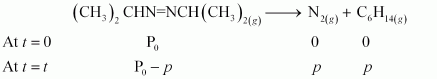After time, t, total pressure, Pt = (Pº - p) + p + p

⇒ Pt = (Pº + p)

⇒ p =
Pt  - P0

therefore, Pº - p = P0  - Pt  - P0

= 2P0 − Pt

For a first order reaction,

k = 2.303/t  Log P0 /P0  - p

=   2.303/t  Log
P0 / 2 P0  -  Pt

When t = 360 s, k = 2.303 / 360s log 35.0 / 2x35.0 - 54.0

= 2.175 × 10−3 s−1

When t = 720 s, k = 2.303 / 720s log 35.0 / 2x35.0 - 63.0

= 2.235 × 10−3 s−1

Hence, the average value of rate constant is

k = (2.175 × 10 - 3  + 2.235 × 10 - 3 ) / 2   s - 1

= 2.21 × 10−3 s−1

Question30. The following data were obtained during the first order thermal decomposition of SO2Cl2 at a constant volume.

SO2Cl2(g)  →  SO2(g) + Cl2(g)

 Experiment Time/s−1 Total pressure/atm 1 0 0.5 2 100 0.6

Calculate the rate of the reaction when total pressure is 0.65 atm.

Solution :
The thermal decomposition of SO2Cl2 at a constant volume is represented by the following equation.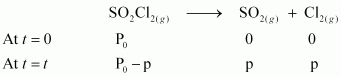After time, t, total pressure, Pt = (Pº - p) + p + p

⇒ Pt = (Pº + p)

⇒ p = Pt  - Pº

therefore, Pº - p = Pº  - Pt  - Pº

= 2 Pº -  Pt

For a first order reaction,

k = 2.303/t  Log  Pº / Pº  - p

=   2.303/t  Log  Pº / 2 Pº  -  Pt

When t= 100 s,

k = 2.303 / 100s log 0.5 / 2x0.5 - 0.6

= 2.231 × 10 - 3s - 1

When Pt= 0.65 atm,

P0+ p= 0.65

⇒ p= 0.65 - P0

= 0.65 - 0.5

= 0.15 atm

Therefore, when the total pressure is 0.65 atm, pressure of SOCl2 is

PSOCL2 = P0 - p

= 0.5 - 0.15

= 0.35 atm

Therefore, the rate of equation, when total pressure is 0.65 atm, is given by,

Rate = k(pSOCL2)

= (2.23 × 10 - 3s - 1) (0.35 atm)

= 7.8 × 10 - 4 atm s - 1

Question31. The rate constant for the decomposition of N2O5 at various temperatures is given below:

 T/°C 0 20 40 60 80 105 X K /S-1 0.0787 1.7 25.7 178 2140

Draw a graph between ln k and 1/T and calculate the values of A and Ea.

Predict the rate constant at 30º and 50ºC.

Solution :
From the given data, we obtain

 T/°C 0 20 40 60 80 T/K 273 293 313 333 353 1/T / k-1 3.66×10−3 3.41×10−3 3.19×10−3 3.0×10−3 2.83 ×10−3 105 X K /S-1 0.0787 1.70 25.7 178 2140 ln k −7.147 − 4.075 −1.359 −0.577 3.063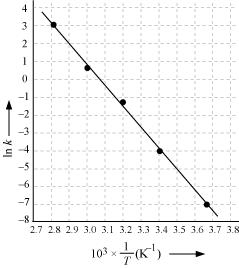Slope of the line,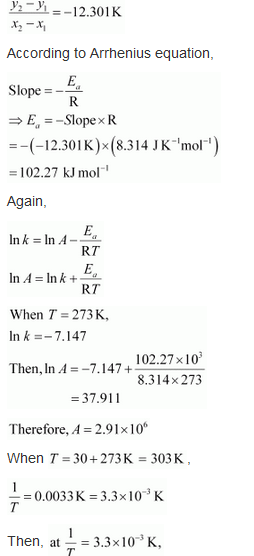In k= - 2.8

Therefore, k = 6.08x10-2s-1

Again when T = 50 + 273K = 323K,

1/T = 3.1 x 10-3 K

In k = - 0.5

Therefore, k = 0.607 s-1

Question32. The rate constant for the decomposition of hydrocarbons is 2.418 × 10−5 s−1 at 546 K. If the energy of activation is 179.9 kJ/mol, what will be the value of pre-exponential factor.

Solution :
k = 2.418 × 10−5 s−1

T = 546 K

Ea = 179.9 kJ mol−1 = 179.9 × 103 J mol−1

According to the Arrhenius equation,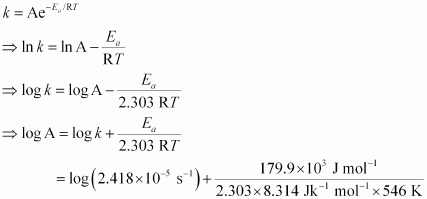= (0.3835 − 5) + 17.2082

= 12.5917

Therefore, A = antilog (12.5917)

= 3.9 × 1012 s−1 (approximately)

Question33. Consider a certain reaction A → Products with k = 2.0 × 10−2 s−1. Calculate the concentration of A remaining after 100 s if the initial concentration of A is 1.0 mol L−1.

Solution :
k = 2.0 × 10−2 s−1

T = 100 s

[A]o = 1.0 moL−1

Since the unit of k is s−1, the given reaction is a first order reaction.

Therefore, k = 2.303/t  Log  [A]º / [A]

⇒2.0 × 110-2 s-1  = 2.303/100s  Log  1.0 / [A]

⇒2.0 × 110-2 s-1  = 2.303/100s  ( - Log [A] )

⇒ - Log [A] = -  (2.0 x 10-2 x 100) /   2.303

⇒ [A] = antilog [-  (2.0 x 10-2 x 100)  /  2.303]

= 0.135 mol L−1 (approximately)

Hence, the remaining concentration of A is 0.135 mol L−1.

Question34. Sucrose decomposes in acid solution into glucose and fructose according to the first order rate law, with t1/2 = 3.00 hours. What fraction of sample of sucrose remains after 8 hours?

Solution :For a first order reaction,

k = 2.303/t  Log  [R]º / [R]

It is given that, t1/2 = 3.00 hours

Therefore, k = 0.693 / t1/2

= 0.693 / 3  h-1

= 0.231 h - 1

Then, 0.231 h - 1 = 2.303 / 8h  Log  [R]º / [R]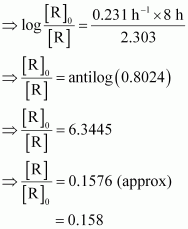Hence, the fraction of sample of sucrose that remains after 8 hours is 0.158.

Question35. The decomposition of hydrocarbon follows the equation k = (4.5 × 1011 s−1) e−28000 K/T Calculate Ea.

Solution :
The given equation is

k = (4.5 × 1011 s−1) e−28000 K/T (i)

Arrhenius equation is given by,

k= Ae -Ea/RT  (ii)

From equation (i) and (ii), we obtain

Ea  / RT  =  28000K / T

⇒ Ea  = R x 28000K

= 8.314 J K−1 mol−1 × 28000 K

= 232792 J mol−1

= 232.792 kJ mol−1

Question36. The rate constant for the first order decomposition of H2O2 is given by the following equation:

log k = 14.34 − 1.25 × 104 K/T

Calculate Ea for this reaction and at what temperature will its half-period be 256 minutes?

Solution :
Arrhenius equation is given by,

k= Ae -Ea/RT

⇒In k = In A - Ea/RT

⇒In k = Log A - Ea/RT

⇒ Log k = Log A - Ea/2.303RT         (i)

The given equation is

Log k = 14.34 - 1.25 104 K/T             (ii)

From equation (i) and (ii), we obtain

Ea/2.303RT  = 1.25 104 K/T

= 1.25 × 104 K × 2.303 × 8.314 J K−1 mol−1

= 239339.3 J mol−1 (approximately)

= 239.34 kJ mol−1

Also, when t1/2 = 256 minutes,

k = 0.693 / t1/2

= 0.693 / 256

= 2.707 × 10 - 3 min - 1

= 4.51 × 10 - 5s - 1

= 2.707 × 10−3 min−1

= 4.51 × 10−5 s−1

It is also given that, log k = 14.34 − 1.25 × 104 K/T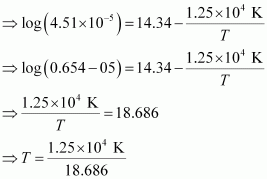= 668.95 K

= 669 K (approximately)

Question37. The decomposition of A into product has value of k as 4.5 × 103 s−1 at 10°C and energy of activation 60 kJ mol−1. At what temperature would k be 1.5 × 104 s−1?

Solution :
From Arrhenius equation, we obtain

log k2/k1 = Ea / 2.303 R (T2 - T1) / T1T2

Also, k1 = 4.5 × 103 s−1

T1 = 273 + 10 = 283 K

k2 = 1.5 × 104 s−1

Ea = 60 kJ mol−1 = 6.0 × 104 J mol−1

Then,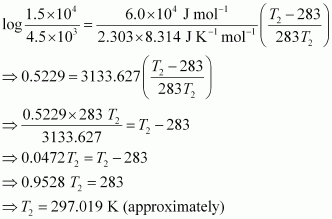= 297 K

= 24°C

Hence, k would be 1.5 × 104 s−1 at 24°C.

Question38. The time required for 10% completion of a first order reaction at 298 K is equal to that required for its 25% completion at 308 K. If the value of A is 4 × 1010 s−1. Calculate k at 318 K and Ea.

Solution :
For a first order reaction,

t = 2.303 / k log a  / a - x

At 298 K, t = 2.303 / k log 100  / 90

= 0.1054 / k

At 308 K, t' = 2.303 / k' log 100  / 75

= 2.2877 / k'

According to the question,

t = t'

0.1054 / k  =  2.2877 / k'

⇒ k' k  = 2.7296

From Arrhenius equation, we obtain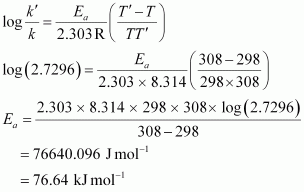To calculate k at 318 K,

It is given that, A = 4 x 1010 s-1, T = 318K

Again, from Arrhenius equation, we obtain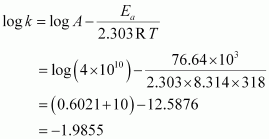Therefore, k = Antilog (-1.9855)

= 1.034 x 10-2 s -1

Question39. The rate of a reaction quadruples when the temperature changes from 293 K to 313 K. Calculate the energy of activation of the reaction assuming that it does not change with temperature.

Solution :
From Arrhenius equation, we obtain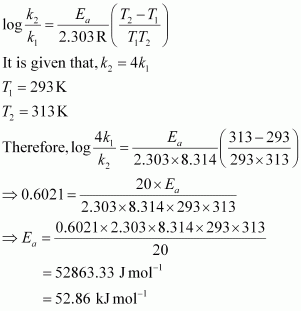Hence, the required energy of activation is 52.86 kJmol−1.

## NCERT Solutions For Class 12 Chemistry Chapter Wise.

Question4. The conversion of molecules X to Y follows second order kinetics. If concentration of X is increased to three times how will it affect the rate of formation of Y?

Solution :
The reaction X → Y follows second order kinetics.

Therefore, the rate equation for this reaction will be:

Rate = k[X]2 (1)

Let [X] = a mol L−1, then equation (1) can be written as:

Rate1 = k .(a)2

= ka2

If the concentration of X is increased to three times, then [X] = 3a mol L−1

Now, the rate equation will be:

Rate = k (3a)2

= 9(ka2)

Hence, the rate of formation will increase by 9 times.

Question5. A first order reaction has a rate constant 1.15 10−3 s−1. How long will 5 g of this reactant take to reduce to 3 g?

Solution :
From the question, we can write down the following information:

Initial amount = 5 g

Final concentration = 3 g

Rate constant = 1.15 10−3 s−1

We know that for a 1st order reaction,= 444.38 s

= 444 s (approx)

Question6. Time required to decompose SO2Cl2 to half of its initial amount is 60 minutes. If the decomposition is a first order reaction, calculate the rate constant of the reaction.

Solution :
We know that for a 1st order reaction,

t½  = 0.693 / k

It is given that t1/2 = 60 min

k = 0.693 / t½

= 0.693 / 60

= 0.01155 min-1

= 1.155 min-1

Or

k = 1.925 x 10-2 s-1

Question7. What will be the effect of temperature on rate constant?

Solution :
The rate constant of a reaction is nearly doubled with a 10° rise in temperature. However, the exact dependence of the rate of a chemical reaction on temperature is given by Arrhenius equation,

k = Ae - Ea / RT

Where,

A is the Arrhenius factor or the frequency factor

T is the temperature

R is the gas constant

Ea is the activation energy

Question8. The rate of the chemical reaction doubles for an increase of 10 K in absolute temperature from 298 K. Calculate Ea.

Solution :
It is given that T1 = 298 K

∴T2 = (298 + 10) K

= 308 K

We also know that the rate of the reaction doubles when temperature is increased by 10°.

Therefore, let us take the value of k1 = k and that of k2 = 2k

Also, R = 8.314 J K−1 mol−1

Now, substituting these values in the equation:= 52897.78 J mol−1

= 52.9 kJ mol−1

Question9. The activation energy for the reaction 2HI(g) → H2 + I2(g) is 209.5 kJ mol−1 at 581K. Calculate the fraction of molecules of reactants having energy equal to or greater than activation energy?

Solution :
In the given case:

Ea = 209.5 kJ mol−1 = 209500 J mol−1

T = 581 K

R = 8.314 JK−1 mol−1

Now, the fraction of molecules of reactants having energy equal to or greater than activation energy is given as:

x = e−Ea / RT

⇒Inx= −Ea / RT

⇒logx=−Ea / 2.303RT

⇒logx= −209500Jmol−1 / 2.303 × 8.314JK−1mol−1×581

=−18.8323

Now,x= Antilog (−18.8323)

=1.471×10−19

Question10. From the rate expression for the following reactions, determine their order of reaction and the dimensions of the rate constants.

(i) 3 NO(g) → N2O (g) Rate = k[NO]2

(ii) H2O2 (aq) + 3 I (aq) + 2 H+ → 2 H2O (l) + I3- Rate = k[H2O2][I]

(iii) CH3CHO(g) → CH4(g) + CO(g) Rate = k [CH3CHO]3/2

(iv) C2H5Cl(g) → C2H4(g) + HCl(g) Rate = k [C2H5Cl]

Solution :
(i) Given rate = k [NO]2

Therefore, order of the reaction = 2

Dimension of  k = Rate / [NO]2

= mol L-1 s-1 / (mol L-1)2

= mol L-1 s
-1 / mol2 L-2

= L mol-1s-1

(ii) Given rate = k [H2O2] [I]

Therefore, order of the reaction = 2

Dimension of

k = Rate  / [H2O2][I - ]

= mol L-1 s-1  / (mol L-1) (mol L-1)

= L mol-1 s-1

(iii) Given rate = k [CH3CHO]3/2

Therefore, order of reaction = 3 / 2

Dimension of  k = Rate / [CH3CHO]3/2

=  mol L-1 s-1   / (mol L-1)3/2

= mol L
-1   s-1     / mol3/2  L-3/2

= L½ mol-½  s-1

(iv) Given rate = k [C2H5Cl]

Therefore, order of the reaction = 1

Dimension of k = Rate /  [C2H5Cl]

= mol L-1 s-1   / mol L-1

= s-1

Question11. For the reaction:

2A + B → A2B

the rate = k[A][B]2 with k = 2.0 × 10−6 mol−2 L2 s−1. Calculate the initial rate of the reaction when [A] = 0.1 mol L−1, [B] = 0.2 mol L−1. Calculate the rate of reaction after [A] is reduced to 0.06 mol L−1.

Solution :
The initial rate of the reaction is

Rate = k [A][B]2

= (2.0 × 10−6 mol−2 L2 s−1) (0.1 mol L−1) (0.2 mol L−1)2

= 8.0 × 10−9 mol−2 L2 s−1

When [A] is reduced from 0.1 mol L−1 to 0.06 mol−1, the concentration of A reacted = (0.1 − 0.06) mol L−1 = 0.04 mol L−1

Therefore, concentration of B reacted 1/2 x 0.04 mol L-1 = 0.02 mol L−1

Then, concentration of B available, [B] = (0.2 − 0.02) mol L−1

= 0.18 mol L−1

After [A] is reduced to 0.06 mol L−1, the rate of the reaction is given by,

Rate = k [A][B]2

= (2.0 × 10−6 mol−2 L2 s−1) (0.06 mol L−1) (0.18 mol L−1)2

= 3.89 mol L−1 s−1

Question12. The decomposition of NH3 on platinum surface is zero order reaction. What are the rates of production of N2 and H2 if k = 2.5 × 10−4 mol−1 L s−1?

Solution :
The decomposition of NH3 on platinum surface is represented by the following equation.= 7.5 × 10−4 mol L−1 s−1

Question13. The decomposition of dimethyl ether leads to the formation of CH4, H2 and CO and the reaction rate is given by

Rate = k [CH3OCH3]3/2

The rate of reaction is followed by increase in pressure in a closed vessel, so the rate can also be expressed in terms of the partial pressure of dimethyl ether, i.e.,

rate = k (PCH3OCH3)3/2

If the pressure is measured in bar andtime in minutes, then what are the units of rate and rate constants?

Solution :
If pressure is measured in bar and time in minutes, then

Unit of rate = bar min−1

Rate = k [CH3OCH3]3/2

⇒ k =Rate / [CH3OCH3]3/2

Therefore, unit of rate constants(k) = bar min−1 / bar3/2

= bar-½ min -1

Question14. Mention the factors that affect the rate of a chemical reaction.

Solution :
The factors that affect the rate of a reaction are as follows.

(i) Concentration of reactants (pressure in case of gases)

(ii) Temperature

(iii) Presence of a catalyst

15. A reaction is second order with respect to a reactant. How is the rate of reaction affected if the concentration of the reactant is

(i) doubled (ii) reduced to half?

Solution :
Letthe concentration of the reactant be [A] = a

Rate of reaction, R = k [A]2

= ka2

(i)If the concentration of the reactant is doubled, i.e. [A] = 2a, then the rate of the reaction would be

R = k(2a)2

= 4ka2

= 4 R

Therefore, the rate of the reaction would increase by 4 times.

(ii) If the concentration of the reactant is reduced to half, i.e. [A] = 1/2 a , then the rate of the reaction would be

R = k(1/2a)2

= 1/4 Ka2

= 1/4 R

Therefore, the rate of the reaction would be reduced to

16. What change would happen in the rate constant of a reaction when there is a change in its temperature? How can this temperature effect on rate constant be represented quantitatively?

Solution :
When a temperature of 10∘ rises for a chemical reaction then the rate constant increases and becomes near to double of its original value.

The temperature effect on the rate constant can be represented quantitatively by Arrhenius equation,

k=Ae−Ea/RT

Where,

k = rate constant,

A = Frequency factor / Arrhenius factor,

R = gas constant

T = temperature

Ea = activation energy for the reaction.

Question17. In a pseudo first order hydrolysis of ester in water, the following results were obtained:

 t/s 0 30 60 90 [Ester]mol L−1 0.55 0.31 0.17 0.085

(i) Calculate the average rate of reaction between the time interval 30 to 60 seconds.

(ii) Calculate the pseudo first order rate constant for the hydrolysis of ester.

Solution :
(i) Average rate of reaction between the time interval, 30 to 60 seconds, d[ester] / dt

= (0.31-0.17) / (60-30)

= 0.14 / 30

= 4.67 × 10−3 mol L−1 s−1

(ii) For a pseudo first order reaction,

k = 2.303/ t log [R]º / [R]

For t = 30 s, k1

= 1.911 × 10−2 s−1

For t = 60 s, k1 = 2.303/ 30 log 0.55 / 0.31

= 1.957 × 10−2 s−1

For t = 90 s,  k3 = 2.303/ 90 log 0.55 / 0.085

= 2.075 × 10 - 2s - 1

= 2.075 × 10−2 s−1

Then, average rate constant, k = k1 + k2+ k3  / 3

= 1.911 × 10 - 2  + 1.957 × 10 - 2 + 2.075 × 10 - 2 / 3

= 1.981 x 10-2 s - 1

Question18. A reaction is first order in A and second order in B.

(i) Write the differential rate equation.

(ii) How is the rate affected on increasing the concentration of B three times?

(iii) How is the rate affected when the concentrations of both A and B are doubled?

Solution :
(i) The differential rate equation will beQuestion19. In a reaction between A and B, the initial rate of reaction (r0) was measured for different initial concentrations of A and B as given below:

 A/ mol L−1 0.20 0.20 0.40 B/ mol L−1 0.30 0.10 0.05 r0/ mol L−1 s−1 5.07 × 10−5 5.07 × 10−5 1.43 × 10−4

What is the order of the reaction with respect to A and B?

Solution :
Let the order of the reaction with respect to A be x and with respect to B be y.

Therefore,Dividing equation (iii) by (ii), we obtain

= 1.496

= 1.5 (approximately)

Hence, the order of the reaction with respect to A is 1.5 and with respect to B is zero.

Question20. The following results have been obtained during the kinetic studies of the reaction:

2A + B → C + D

 Experiment A/ mol L−1 B/ mol L−1 Initial rate of formation of D/mol L−1 min−1 I 0.1 0.1 6.0 × 10−3 II 0.3 0.2 7.2 × 10−2 III 0.3 0.4 2.88 × 10−1 IV 0.4 0.1 2.40 × 10−2

Determine the rate law and the rate constant for the reaction.

Solution :
Let the order of the reaction with respect to A be x and with respect to B be y.

Therefore, rate of the reaction is given by,Dividing equation (iv) by (i), we obtainDividing equation (iii) by (ii), we obtainQuestion21. The reaction between A and B is first order with respect to A and zero order with respect to B. Fill in the blanks in the following table:

 Experiment A/ mol L−1 B/ mol L−1 Initial rate/mol L−1 min−1 I 0.1 0.1 2.0 × 10−2 II -- 0.2 4.0 × 10−2 III 0.4 0.4 -- IV -- 0.2 2.0 × 10−2

Solution :
The given reaction is of the first order with respect to A and of zero order with respect to B.

Therefore, the rate of the reaction is given by,

Rate = k [A]1 [B]0

⇒ Rate = k [A]

From experiment I, we obtain

2.0 × 10−2 mol L−1 min−1 = k (0.1 mol L−1)

⇒ k = 0.2 min−1

From experiment II, we obtain

4.0 × 10−2 mol L−1 min−1 = 0.2 min−1 [A]

⇒ [A] = 0.2 mol L−1

From experiment III, we obtain

Rate = 0.2 min−1 × 0.4 mol L−1

= 0.08 mol L−1 min−1

From experiment IV, we obtain

2.0 × 10−2 mol L−1 min−1 = 0.2 min−1 [A]

⇒ [A] = 0.1 mol L−1

Question22. Calculate the half-life of a first order reaction from their rate constants given below:

(i) 200 s−1 (ii) 2 min−1 (iii) 4 years−1

Solution :
(i) Half life, t 1/2 = 0.693 / k

= 0.693 / 200 s-1

= 3.47×10 -3 s (approximately)

(ii) Half life, t 1/2 = 0.693 / k

= 0.693 / 2 min-1

= 0.35 min (approximately)

(iii) Half life, t 1/2 = 0.693 / k

= 0.693 / 4 years-1

= 0.173 years (approximately)

Question23. The half-life for radioactive decay of 14C is 5730 years. An archaeological artifact containing wood had only 80% of the 14C found in a living tree. Estimate the age of the sample.

Solution :
Here,  k = 0.693 / t1/2

= 0.693 / 5730 years-1

It is known that,= 1845 years (approximately)

Hence, the age of the sample is 1845 years.

Question24. The experimental data for decomposition of N2O5

[2N2O5 → 4NO2 + O2]  in gas phase at 318K are given below:

 t(s) 0 400 800 1200 1600 2000 2400 2800 3200 102 × [N2O5]  mol L-1 1.63 1.36 1.14 0.93 0.78 0.64 0.53 0.43 0.35

(i) Plot [N2O5] against t.

(ii) Find the half-life period for the reaction.

(iii) Draw a graph between log [N2O5] and t.

(iv) What is the rate law?

(v) Calculate the rate constant.

(vi) Calculate the half-life period from k and compare it with (ii).

Solution :(ii) Time corresponding to the concentration, 1630x102 / 2 mol L-1 = 81.5 mol L-1 is the half life. From the graph, the half life is obtained as 1450 s.

(iii)

 t(s) 102 × [N2O5]  mol L-1 Log[N2O5] 0 1.63 − 1.79 400 1.36 − 1.87 800 1.14 − 1.94 1200 0.93 − 2.03 1600 0.78 − 2.11 2000 0.64 − 2.19 2400 0.53 − 2.28 2800 0.43 − 2.37 3200 0.35 − 2.46(iv) The given reaction is of the first order as the plot, Log[N2O5v/s t, is a straight line. Therefore, the rate law of the reaction is

Rate = k [N2O5]

(v) From the plot, Log[N2O5] v/s t, we obtain

- k /2.303

Again, slope of the line of the plot  Log[N2O5v/s t is given by

- k / 2.303. = -0.67 / 3200

Therefore, we obtain,

- k / 2.303  = - 0.67 / 3200

⇒ k = 4.82 x 10-4 s-1

(vi) Half-life is given by,

t½ = 0.693 / k

= 0.639 / 4.82x10-4 s

=1.438 x 103

This value, 1438 s, is very close to the value that was obtained from the graph.

25. The rate constant for a first order reaction is 60 s−1. How much time will it take to reduce the initial concentration of the reactant to its 1/16th value?

Solution :
It is known that,Question26. During nuclear explosion, one of the products is 90Sr with half-life of 28.1 years. If 1μg of 90Sr was absorbed in the bones of a newly born baby instead of calcium, how much of it will remain after 10 years and 60 years if it is not lost metabolically.

Solution :
,Therefore, 0.7814 μg of 90Sr will remain after 10 years.

Again,Therefore, 0.2278 μg of 90Sr will remain after 60 years.

Question27. For a first order reaction, show that time required for 99% completion is twice the time required for the completion of 90% of reaction.

Solution :
For a first order reaction, the time required for 99% completion is

t1 = 2.303/k Log 100/100-99

= 2.303/k Log 100

= 2x 2.303/k

For a first order reaction, the time required for 90% completion is

t2 = 2.303/k Log 100 / 100-90

= 2.303/k Log 10

= 2.303/k

Therefore, t1 = 2t2

Hence, the time required for 99% completion of a first order reaction is twice the time required for the completion of 90% of the reaction.

Question28. A first order reaction takes 40 min for 30% decomposition. Calculate t1/2.

Solution :
For a first order reaction,

t = 2.303/k Log [R] º / [R]

k = 2.303/40min  Log 100 / 100-30

= 2.303/40min  Log 10 / 7

= 8.918 x 10-3 min-1

Therefore, t1/2 of the decomposition reaction is

t1/2 = 0.693/k

=  0.693 / 8.918 x 10-3  min

= 77.7 min (approximately)

= 77.7 min (approximately)

Question29. For the decomposition of azoisopropane to hexane and nitrogen at 543 K, the following data are obtained.

 t (sec) P(mm of Hg) 0 35.0 360 54.0 720 63.0

Calculate the rate constant.

Solution :
The decomposition of azoisopropane to hexane and nitrogen at 543 K is represented by the following equation.After time, t, total pressure, Pt = (Pº - p) + p + p

⇒ Pt = (Pº + p)

⇒ p =
Pt  - P0

therefore, Pº - p = P0  - Pt  - P0

= 2P0 − Pt

For a first order reaction,

k = 2.303/t  Log P0 /P0  - p

=   2.303/t  Log
P0 / 2 P0  -  Pt

When t = 360 s, k = 2.303 / 360s log 35.0 / 2x35.0 - 54.0

= 2.175 × 10−3 s−1

When t = 720 s, k = 2.303 / 720s log 35.0 / 2x35.0 - 63.0

= 2.235 × 10−3 s−1

Hence, the average value of rate constant is

k = (2.175 × 10 - 3  + 2.235 × 10 - 3 ) / 2   s - 1

= 2.21 × 10−3 s−1

Question30. The following data were obtained during the first order thermal decomposition of SO2Cl2 at a constant volume.

SO2Cl2(g)  →  SO2(g) + Cl2(g)

 Experiment Time/s−1 Total pressure/atm 1 0 0.5 2 100 0.6

Calculate the rate of the reaction when total pressure is 0.65 atm.

Solution :
The thermal decomposition of SO2Cl2 at a constant volume is represented by the following equation.After time, t, total pressure, Pt = (Pº - p) + p + p

⇒ Pt = (Pº + p)

⇒ p = Pt  - Pº

therefore, Pº - p = Pº  - Pt  - Pº

= 2 Pº -  Pt

For a first order reaction,

k = 2.303/t  Log  Pº / Pº  - p

=   2.303/t  Log  Pº / 2 Pº  -  Pt

When t= 100 s,

k = 2.303 / 100s log 0.5 / 2x0.5 - 0.6

= 2.231 × 10 - 3s - 1

When Pt= 0.65 atm,

P0+ p= 0.65

⇒ p= 0.65 - P0

= 0.65 - 0.5

= 0.15 atm

Therefore, when the total pressure is 0.65 atm, pressure of SOCl2 is

PSOCL2 = P0 - p

= 0.5 - 0.15

= 0.35 atm

Therefore, the rate of equation, when total pressure is 0.65 atm, is given by,

Rate = k(pSOCL2)

= (2.23 × 10 - 3s - 1) (0.35 atm)

= 7.8 × 10 - 4 atm s - 1

Question31. The rate constant for the decomposition of N2O5 at various temperatures is given below:

 T/°C 0 20 40 60 80 105 X K /S-1 0.0787 1.7 25.7 178 2140

Draw a graph between ln k and 1/T and calculate the values of A and Ea.

Predict the rate constant at 30º and 50ºC.

Solution :
From the given data, we obtain

 T/°C 0 20 40 60 80 T/K 273 293 313 333 353 1/T / k-1 3.66×10−3 3.41×10−3 3.19×10−3 3.0×10−3 2.83 ×10−3 105 X K /S-1 0.0787 1.70 25.7 178 2140 ln k −7.147 − 4.075 −1.359 −0.577 3.063Slope of the line,In k= - 2.8

Therefore, k = 6.08x10-2s-1

Again when T = 50 + 273K = 323K,

1/T = 3.1 x 10-3 K

In k = - 0.5

Therefore, k = 0.607 s-1

Question32. The rate constant for the decomposition of hydrocarbons is 2.418 × 10−5 s−1 at 546 K. If the energy of activation is 179.9 kJ/mol, what will be the value of pre-exponential factor.

Solution :
k = 2.418 × 10−5 s−1

T = 546 K

Ea = 179.9 kJ mol−1 = 179.9 × 103 J mol−1

According to the Arrhenius equation,= (0.3835 − 5) + 17.2082

= 12.5917

Therefore, A = antilog (12.5917)

= 3.9 × 1012 s−1 (approximately)

Question33. Consider a certain reaction A → Products with k = 2.0 × 10−2 s−1. Calculate the concentration of A remaining after 100 s if the initial concentration of A is 1.0 mol L−1.

Solution :
k = 2.0 × 10−2 s−1

T = 100 s

[A]o = 1.0 moL−1

Since the unit of k is s−1, the given reaction is a first order reaction.

Therefore, k = 2.303/t  Log  [A]º / [A]

⇒2.0 × 110-2 s-1  = 2.303/100s  Log  1.0 / [A]

⇒2.0 × 110-2 s-1  = 2.303/100s  ( - Log [A] )

⇒ - Log [A] = -  (2.0 x 10-2 x 100) /   2.303

⇒ [A] = antilog [-  (2.0 x 10-2 x 100)  /  2.303]

= 0.135 mol L−1 (approximately)

Hence, the remaining concentration of A is 0.135 mol L−1.

Question34. Sucrose decomposes in acid solution into glucose and fructose according to the first order rate law, with t1/2 = 3.00 hours. What fraction of sample of sucrose remains after 8 hours?

Solution :For a first order reaction,

k = 2.303/t  Log  [R]º / [R]

It is given that, t1/2 = 3.00 hours

Therefore, k = 0.693 / t1/2

= 0.693 / 3  h-1

= 0.231 h - 1

Then, 0.231 h - 1 = 2.303 / 8h  Log  [R]º / [R]Hence, the fraction of sample of sucrose that remains after 8 hours is 0.158.

Question35. The decomposition of hydrocarbon follows the equation k = (4.5 × 1011 s−1) e−28000 K/T Calculate Ea.

Solution :
The given equation is

k = (4.5 × 1011 s−1) e−28000 K/T (i)

Arrhenius equation is given by,

k= Ae -Ea/RT  (ii)

From equation (i) and (ii), we obtain

Ea  / RT  =  28000K / T

⇒ Ea  = R x 28000K

= 8.314 J K−1 mol−1 × 28000 K

= 232792 J mol−1

= 232.792 kJ mol−1

Question36. The rate constant for the first order decomposition of H2O2 is given by the following equation:

log k = 14.34 − 1.25 × 104 K/T

Calculate Ea for this reaction and at what temperature will its half-period be 256 minutes?

Solution :
Arrhenius equation is given by,

k= Ae -Ea/RT

⇒In k = In A - Ea/RT

⇒In k = Log A - Ea/RT

⇒ Log k = Log A - Ea/2.303RT         (i)

The given equation is

Log k = 14.34 - 1.25 104 K/T             (ii)

From equation (i) and (ii), we obtain

Ea/2.303RT  = 1.25 104 K/T

= 1.25 × 104 K × 2.303 × 8.314 J K−1 mol−1

= 239339.3 J mol−1 (approximately)

= 239.34 kJ mol−1

Also, when t1/2 = 256 minutes,

k = 0.693 / t1/2

= 0.693 / 256

= 2.707 × 10 - 3 min - 1

= 4.51 × 10 - 5s - 1

= 2.707 × 10−3 min−1

= 4.51 × 10−5 s−1

It is also given that, log k = 14.34 − 1.25 × 104 K/T= 668.95 K

= 669 K (approximately)

Question37. The decomposition of A into product has value of k as 4.5 × 103 s−1 at 10°C and energy of activation 60 kJ mol−1. At what temperature would k be 1.5 × 104 s−1?

Solution :
From Arrhenius equation, we obtain

log k2/k1 = Ea / 2.303 R (T2 - T1) / T1T2

Also, k1 = 4.5 × 103 s−1

T1 = 273 + 10 = 283 K

k2 = 1.5 × 104 s−1

Ea = 60 kJ mol−1 = 6.0 × 104 J mol−1

Then,= 297 K

= 24°C

Hence, k would be 1.5 × 104 s−1 at 24°C.

Question38. The time required for 10% completion of a first order reaction at 298 K is equal to that required for its 25% completion at 308 K. If the value of A is 4 × 1010 s−1. Calculate k at 318 K and Ea.

Solution :
For a first order reaction,

t = 2.303 / k log a  / a - x

At 298 K, t = 2.303 / k log 100  / 90

= 0.1054 / k

At 308 K, t' = 2.303 / k' log 100  / 75

= 2.2877 / k'

According to the question,

t = t'

0.1054 / k  =  2.2877 / k'

⇒ k' k  = 2.7296

From Arrhenius equation, we obtainTo calculate k at 318 K,

It is given that, A = 4 x 1010 s-1, T = 318K

Again, from Arrhenius equation, we obtainTherefore, k = Antilog (-1.9855)

= 1.034 x 10-2 s -1

Question39. The rate of a reaction quadruples when the temperature changes from 293 K to 313 K. Calculate the energy of activation of the reaction assuming that it does not change with temperature.

Solution :
From Arrhenius equation, we obtainHence, the required energy of activation is 52.86 kJmol−1.